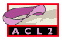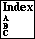## LINEAR-ARITHMETIC

A description of the linear arithmetic decision procedure
```Major Section:  MISCELLANEOUS
```

We describe the procedure very roughly here. Fundamental to the procedure is the notion of a linear polynomial inequality. A ``linear polynomial'' is a sum of terms, each of which is the product of a rational constant and an ``unknown.'' The ``unknown'' is permitted to be `1` simply to allow a term in the sum to be constant. Thus, an example linear polynomial is `3*x + 7*a + 2`; here `x` and `a` are the (interesting) unknowns. However, the unknowns need not be variable symbols. For example, `(length x)` might be used as an unknown in a linear polynomial. Thus, another linear polynomial is `3*(length x) + 7*a`. A ``linear polynomial inequality'' is an inequality (either `<` or `<=`) relation between two linear polynomials. Note that an equality may be considered as a pair of inequalities; e.q., `3*x + 7*a + 2 = 0` is the same as the conjunction of `3*x + 7*a + 2 <= 0` and `0 <= 3*x + 7*a + 2`.

Certain linear polynomial inequalities can be combined by cross-multiplication and addition to permit the deduction of a third inequality with fewer unknowns. If this deduced inequality is manifestly false, a contradiction has been deduced from the assumed inequalities.

For example, suppose we have two assumptions

```p1:       3*x + 7*a <  4
p2:               3 <  2*x
```
and we wish to prove that, given `p1` and `p2`, `a < 0`. As suggested above, we proceed by assuming the negation of our goal
```p3:               0 <= a.
```
and looking for a contradiction.

By cross-multiplying and adding the first two inequalities, (that is, multiplying `p1` by `2`, `p2` by `3` and adding the respective sides), we deduce the intermediate result

```p4:  6*x + 14*a + 9 < 8 + 6*x
```
which, after cancellation, is:
```p4:        14*a + 1 <  0.
```
If we then cross-multiply and add `p3` to `p4`, we get
```p5:               1 <= 0,
```
a contradiction. Thus, we have proved that `p1` and `p2` imply the negation of `p3`.

All of the unknowns of an inequality must be eliminated by cancellation in order to produce a constant inequality. We can choose to eliminate the unknowns in any order, but we eliminate them in term-order, largest unknowns first. (See term-order.) That is, two polys are cancelled against each other only when they have the same largest unknown. For instance, in the above example we see that `x` is the largest unknown in each of `p1` and `p2`, and `a` in `p3` and `p4`.

Now suppose that this procedure does not produce a contradiction but instead yields a set of nontrivial inequalities. A contradiction might still be deduced if we could add to the set some additional inequalities allowing further cancellations. That is where `:linear` lemmas come in. When the set of inequalities has stabilized under cross-multiplication and addition and no contradiction is produced, we search the data base of `:``linear` rules for rules about the unknowns that are candidates for cancellation (i.e., are the largest unknowns in their respective inequalities). See linear for a description of how `:``linear` rules are used.

See also `non-linear-arithmetic` for a description of an extension to the linear-arithmetic procedure described here.